How to use SMALL function in Microsoft Excel

In Excel, SMALL function is used to return the k’th smallest value from an array or a range of numerical values.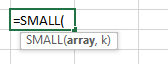Let’s take examples and understand:

We have taken 7 different examples for the usage of SMALL function in Microsoft Excel on the basis of following sample data.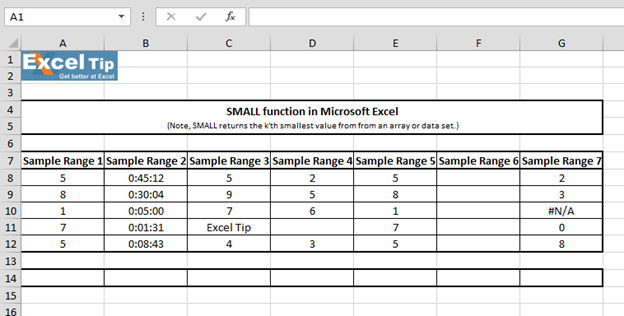1st Example:-

In this example, we will learn how to find out the smallest number within a range.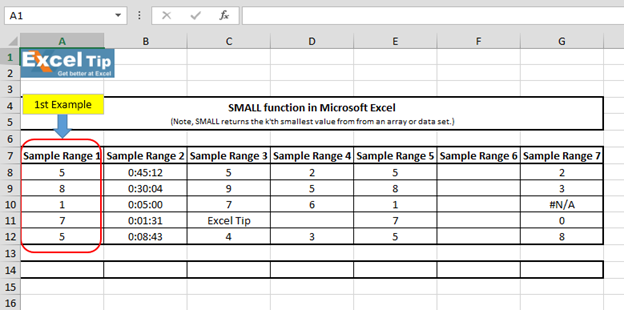• Enter the function in cell A14
• =SMALL(A8:A12,1)
• Press Enter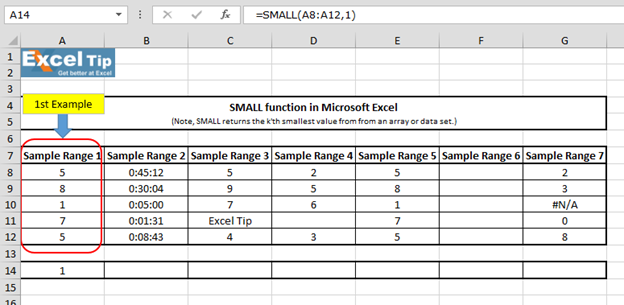The function has given 1. SMALL function takes two arguments, where first argument is an array and the second is the k’th value.

2nd Example:-

In this example, we will learn how we can find out the 2nd smallest time value.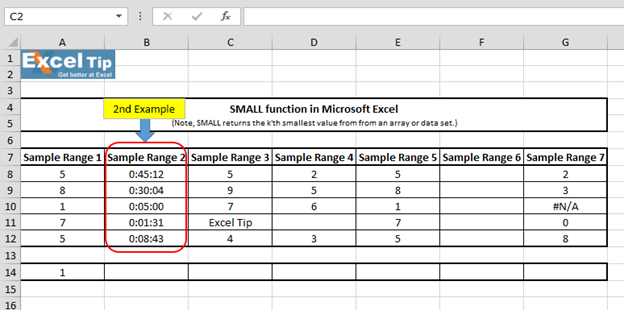• Enter the function in cell B14
• =SMALL(B8:B12,2), Press Enter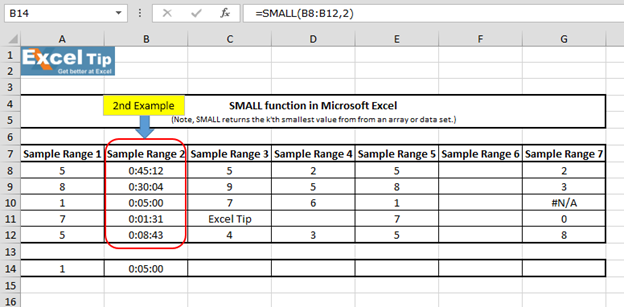The function has returned 0:05:00, which is 2nd smallest value in the defined range.

Note: To get the smallest number according to different position, we have to change the kth value’s number.

3rd Example:-

In this example, we have included text in the range for which we want to return the smallest value.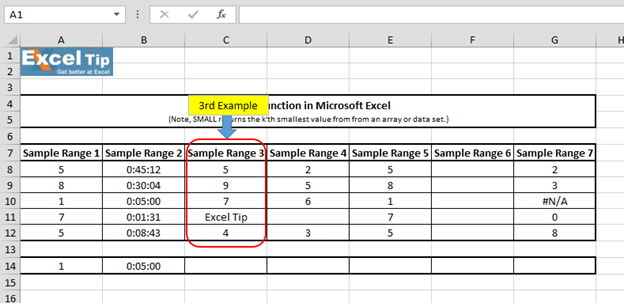• Enter the function in cell C14
• =SMALL(C8:C12,1)
• Press Enter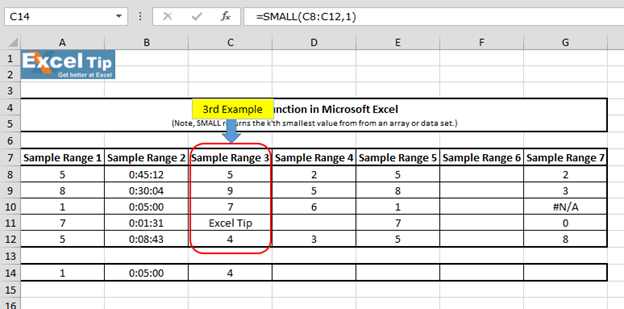The function has returned 4, which is the 1st smallest value within a range. It means function will ignore the cell which contains text and consider cells that contain numbers value only and returns 4, which is the smallest value in the range.

4th Example:-

In this example, we will see what happens if range contains blank cells from which we want to return 2nd smallest value.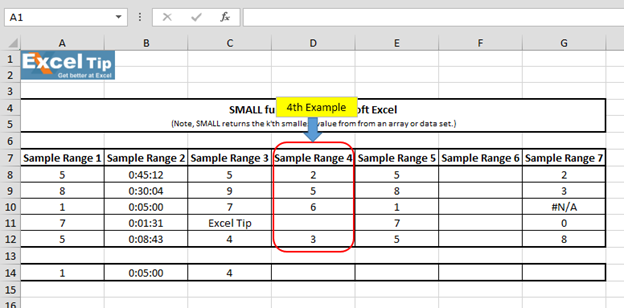• Enter the function in cell D14
• =SMALL(D8:D12,2), Press Enter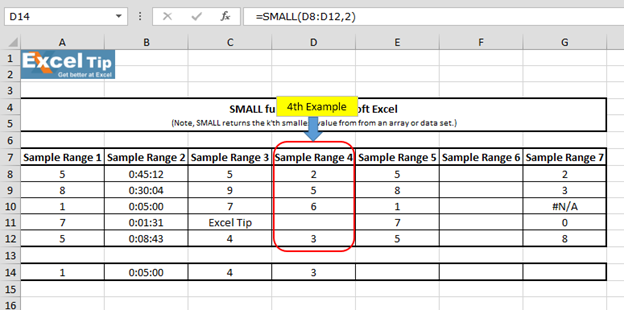Function returns the 2nd smallest value from the entered range. And, as we hit enter we get 3, which is the 2nd smallest value in the range. It means SMALL function ignores blank cells as well.

5th Example:-

In this example, we will see what happens if we enter k’th value larger than the number of data points in array.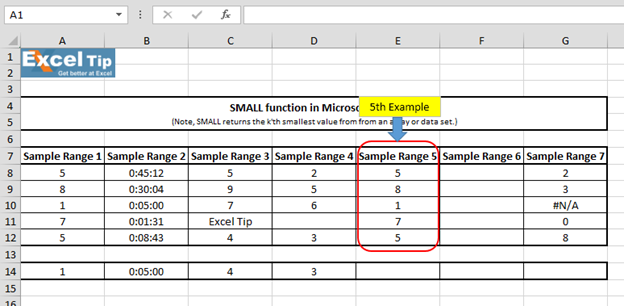• Enter the function in cell E14
• =SMALL(E8:E12,7), Press Enter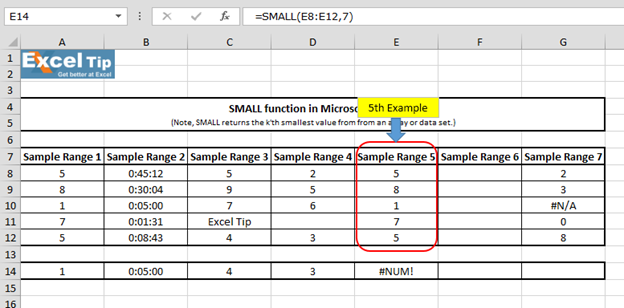Function has returned #NUM error. It is because K’th value should not lie above the data point’s number or else it will return #NUM error.

6th Example:-

In this example, we will see what happens when we enter the SMALL function for blank range.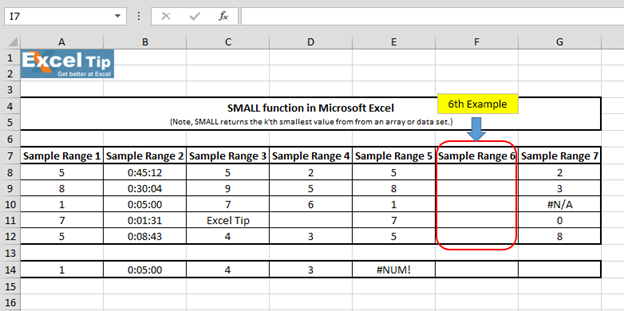• Enter the function in cell F14
• =SMALL(F8:F12,1), Press Enter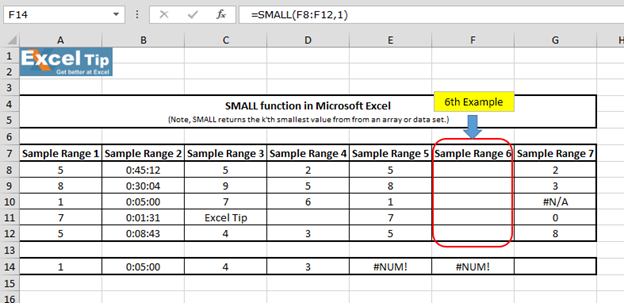It gives us #NUM error again because both arguments are a must and cannot be empty, which is why function returned an error here.

7th Example:-

In this example, we will see what result we get when our defined range contains any error value.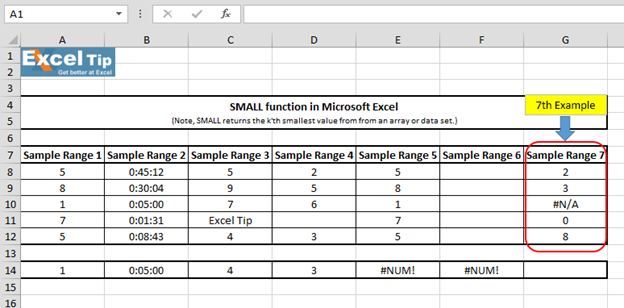• Enter the function in cell G14
• =SMALL(G8:G12,1), Press Enter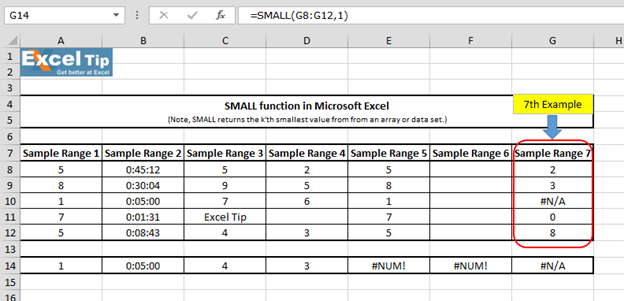The function has returned the #N/A error because there is #N/A error in the range.

So, this is how SMALL function works, and we can make use of it in different situations.# Video: How to use SMALL function in Microsoft Excel

Click on the video link for quick reference to the use of SMALL function. Subscribe to our new channel and keep learning with us!